## Want to keep learning?

This content is taken from the The Open University & Persontyle's online course, Advanced Machine Learning. Join the course to learn more.
1.12

# Expected Loss, the Bias-Variance Decompostion & Overfitting

We will discuss the idea that training a statistical model is equivalent to finding values for its parameters that optimize a loss function. In doing this, we will be able to discover the value for the loss function on the training data.

It is important to understand, though, that optimizing the performance of the model on the training data is not our goal. What we normally want is, rather, to optimize the performance of the model on new data. This is the expected loss on new data.

#### Aside: Transductive Learning

Very occasionally it will be of interest to us that it do well only on particular set of new data. In such a case the expected loss of the model on all possible data loses importance. Instead we want to know the expected loss on that particular set of new data.

Before considering what this desire to minimize expected loss on new data means for us when training a model, let us first analyze the expect loss, here after the expected error, of a model on data.

To do this, we introduce three concepts:

1. The Irreducible Error. This is the inherent randomness in the system being modelled.
2. The Bias of the Model. This is the extent to which the expected value of the system differs from the expected value of the model.
3. The Variance of the Model. This is just what is stated: The variance of the model function. Intuitively it can be understood as the extent to which the model moves around its mean.

The bias-variance decomposition is the decomposition of the expected error of a model into these three concepts, with the formula:

$Expected Error = Irreducible Error + Variance + Bias^2$

Interested students can see a formal derivation of the bias-variance decomposition in the Deriving the Bias Variance Decomposition document available in the related links at the end of the article.

Since there is nothing we can do about irreducible error, our aim in statistical learning must be to find models than minimize variance and bias. Now consider the ‘sophistication’ or ‘complexity’ of a function, considered informally as simply it’s curviness. Consider, for example, the curves on the following image: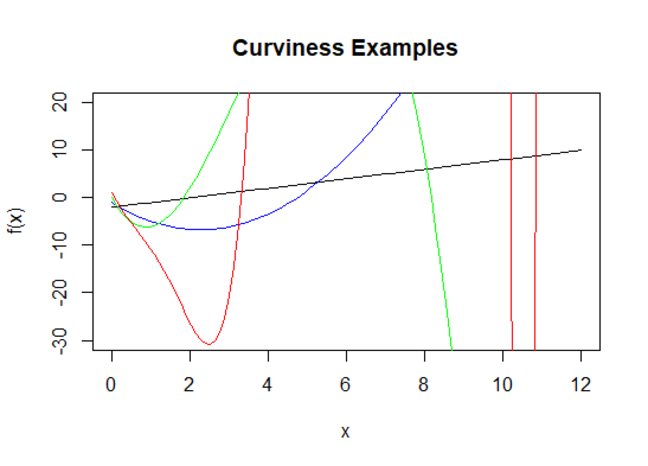The curves in this example are:

Black: $$-2+x$$

Blue: $$-1-5x+1.1x^2$$

Green: $$15x+10x^2-x^3$$

Red: $$1-15x+5x^2-3x^4+x^5-.01x^7+.000003*x^{10}$$

Clearly in terms of curviness Black < Blue < Green < Red, and so we specify that whatever complexity is, the same is true of it.

Simple models will struggle to model complex functions. Consider a very curvy function being modeled by a linear function. No matter how hard we seek to model this curvy function, our linear model will never get it exactly right. It is, we would say, biased. An example is given below: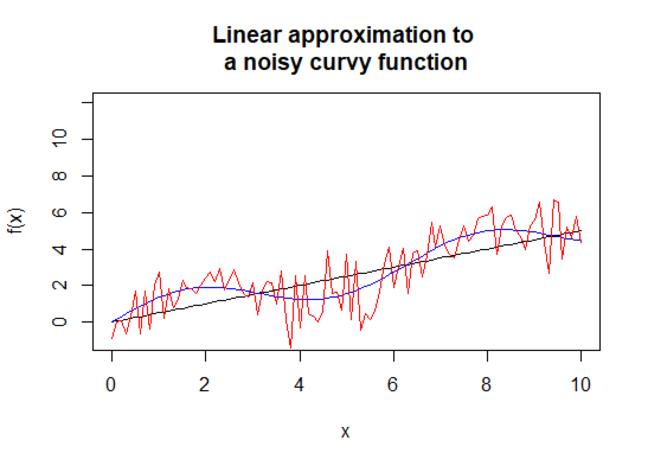Here the red curve is the true function (which includes noise). The blue curve is the deterministic component of the function, and the black curve is our linear approximation. This is a good example not only of the inability of the linear model to accurately model a curvy function, but also of irreducible error: No model can do better than the blue curve since the difference between the red and blue curves is due entirely to random noise.

As the models we use are allowed to get more complex (more curvy in our discussion) they will be able to model more and more real world systems with reasonable degrees of accuracy.

Intuitively, however, as a function gets more complex (curvy) it will ‘move around’ more too. And so we might expect that its variance will increase. This is, in fact, exactly what does occur. Accordingly, we can envision the components of expected error as a function of complexity, when irreducible error stays constant, bias reduces and variance increases.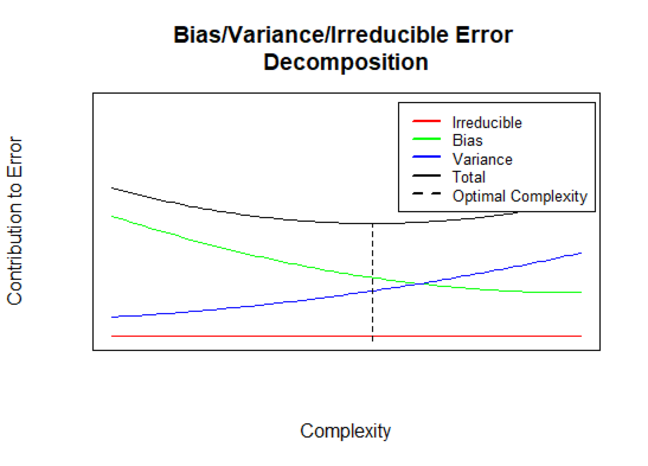This graph tells us that we cannot adjust the complexity of a model to both reduce bias and variance simultaneously: In seeking an optimal model we seek the best trade-off between bias and variance. Notice that the optimal point is not necessarily where the bias and variance curves cross.

It must be emphasised that the graph shown holds fixed the amount of training data. It is possible to reduce both bias and variance by increase the amount of training data used, and often altering the complexity of the model as the training data is increased is essential to finding the optimal model.

A common, though imperfect, measure of the complexity of a statistical model is the number of parameters it has. We will adopt such a definition here (though we will have reason to adjust it later).

## Overfitting

When we utilize a model that is too complex for the function being modelled (given the amount of learning data we have available) we see that the variance component of the expected error increases. In popular parlance this is known as overfitting.

It is important to note that increasing the complexity of a model will always lead to better performance on the training data. Essentially, by increasing complexity (increasing the number of parameters that can be fit to the training data) we increase the ability of the model to customize itself to the training data. This is great if what it is doing is fitting itself to patterns in the training data that are present in the entire population (i.e. in all data). But it is not good if what is actually going on is that the model is fitting itself to random noise present only in the training data. An example is given in the following graph: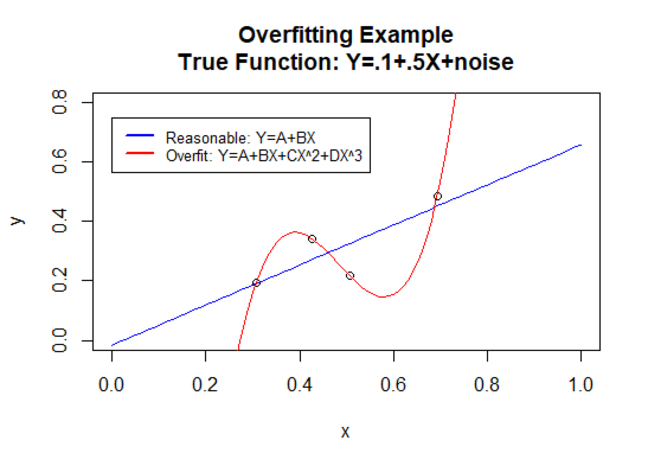The blue line is a linear regression (OLS) model. The red is a polynomial regression model of third order. The form of these models (written more clearly than in the legend) is:

Linear Regression Model: $$\hat y=\beta_0 + \beta_1x$$

Polynomial Regression Model: $$\hat y=\beta_0 + \beta_1x+ \beta_2x^2+ \beta_3x^3$$

Note that the linear model has two parameters and the polynomial model has four. These models have been fit to minimize the MSE loss function on the training data. Clearly the polynomial model has a lower MSE on the training data. In fact it has no MSE - it fits the data perfectly. But looking at the true function we know that it will perform worse on new data. It will not generalize well. It has overfitted the training data.

To emphasise the fact that complexity causing overfitting is related to the amount of training data, examine the effect of training the two models on 100 points instead of 4: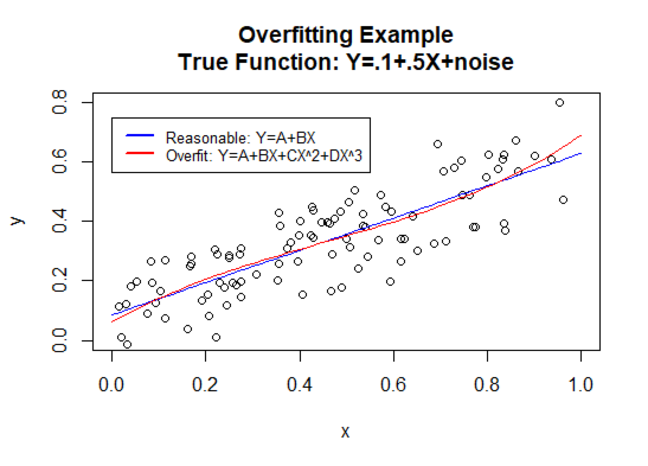Overfitting is one of the most constant dangers of advanced statistical machine larning. The models we work with tend to be very complex, and so able to overfit even when trained of reasonably large sets of training data.

How do we choose models that have low expected error? We will have discuss this in detail in week 2. But already it should be obvious that one thing we can do is see how models perform and data that they have not been trained on. This is the basis of validation techniques for model evaluation and selection.